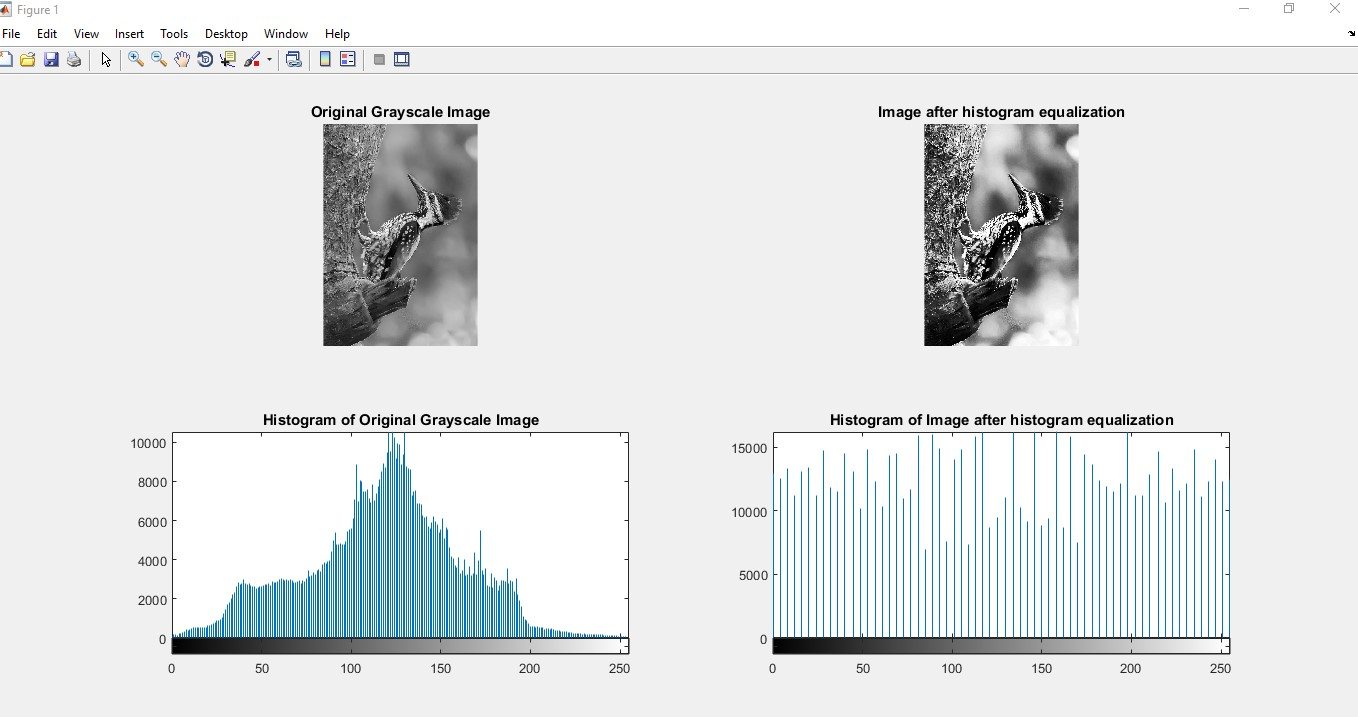Impact-Site-Verification: dbe48ff9-4514-40fe-8cc0-70131430799e

## Search This Blog

Histogram Equalization

The process of adjusting intensity values can be done automatically using histogram equalization. Histogram equalization involves transforming the intensity values so that the histogram of the output image approximately matches a specified histogram. By default, the histogram equalization function, histeq, tries to match a flat histogram with 64 bins, but you can specify a different histogram instead.

Notice how this curve reflects the histograms in the previous figure, with the input values mostly between 0.3 and 0.6, while the output values are distributed evenly between 0 and 1.

% Code:

clc

clear all

close all

b=rgb2gray(a);

subplot(2,2,1);

imshow(b);

title('Original Grayscale Image');

subplot(2,2,3);

imhist(b);

title('Histogram of Original Grayscale Image');

j=histeq(b);

subplot(2,2,2);

imshow(j);

title('Image after histogram equalization');

subplot(2,2,4);

imhist(j);

title('Histogram of Image after histogram equalization');

Original Image: taken from https://www.lenshorizon.com/Grey Scale Image with Histogram